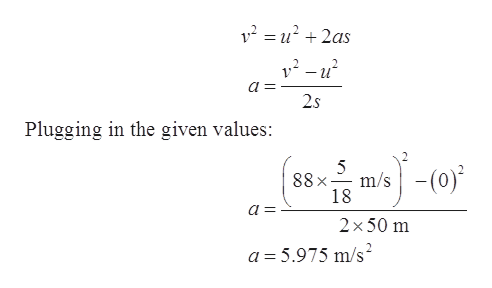# The cheetah can reach a top speed of 114 km/h (71 mi/h). While chasing its prey in a short sprint, a cheetah starts from rest and runs 50 m in a straight line, reaching a final speed of 88 km/h.(a) Determine the cheetah's average acceleration during the short sprint. (b) Find its displacement at t = 3.3 s. (Assume the cheetah maintains a constant acceleration throughout the sprint.)

Question
18 views

The cheetah can reach a top speed of 114 km/h (71 mi/h). While chasing its prey in a short sprint, a cheetah starts from rest and runs 50 m in a straight line, reaching a final speed of 88 km/h.

(a) Determine the cheetah's average acceleration during the short sprint.

(b) Find its displacement at

t = 3.3 s.

(Assume the cheetah maintains a constant acceleration throughout the sprint.)

check_circle

Step 1

Given data:

Initial speed of the cheetah, u = 0 km/h

Final speed of the cheetah, v = 88 km/h

Distance covered, s = 50 m

Step 2

Writing the third equation of the kinematics:help_outlineImage Transcriptionclose12u22as v2-u a = 2s Plugging in the given values: 5 88x m/s 18 2 x 50 m a 5.975 m/s2 fullscreen
Step 3

(b)

Let the displacement be s

Writing the se...

### Want to see the full answer?

See Solution

#### Want to see this answer and more?

Solutions are written by subject experts who are available 24/7. Questions are typically answered within 1 hour.*

See Solution
*Response times may vary by subject and question.
Tagged in

### Other